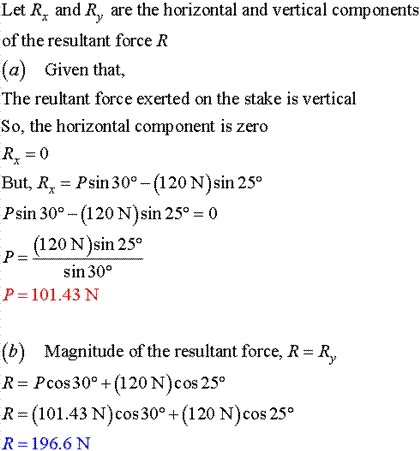# A stake is being pulled out of the ground by means of two ropes as shown. Knowing that α 5 30°, determine by trigonometry (a) the magnitude of the force P so that the resultant force exerted on the stake is vertical, (b) the corresponding magnitude of the resultant.

Question-AnswerCategory: Engineering MechanicsA stake is being pulled out of the ground by means of two ropes as shown. Knowing that α 5 30°, determine by trigonometry (a) the magnitude of the force P so that the resultant force exerted on the stake is vertical, (b) the corresponding magnitude of the resultant.

### A stake is being pulled out of the ground by means of two ropes as shown. Knowing that α = 30°, determine by trigonometry (a) the magnitude of the force P so that the resultant force exerted on the stake is vertical, (b) the corresponding magnitude of the resultant.